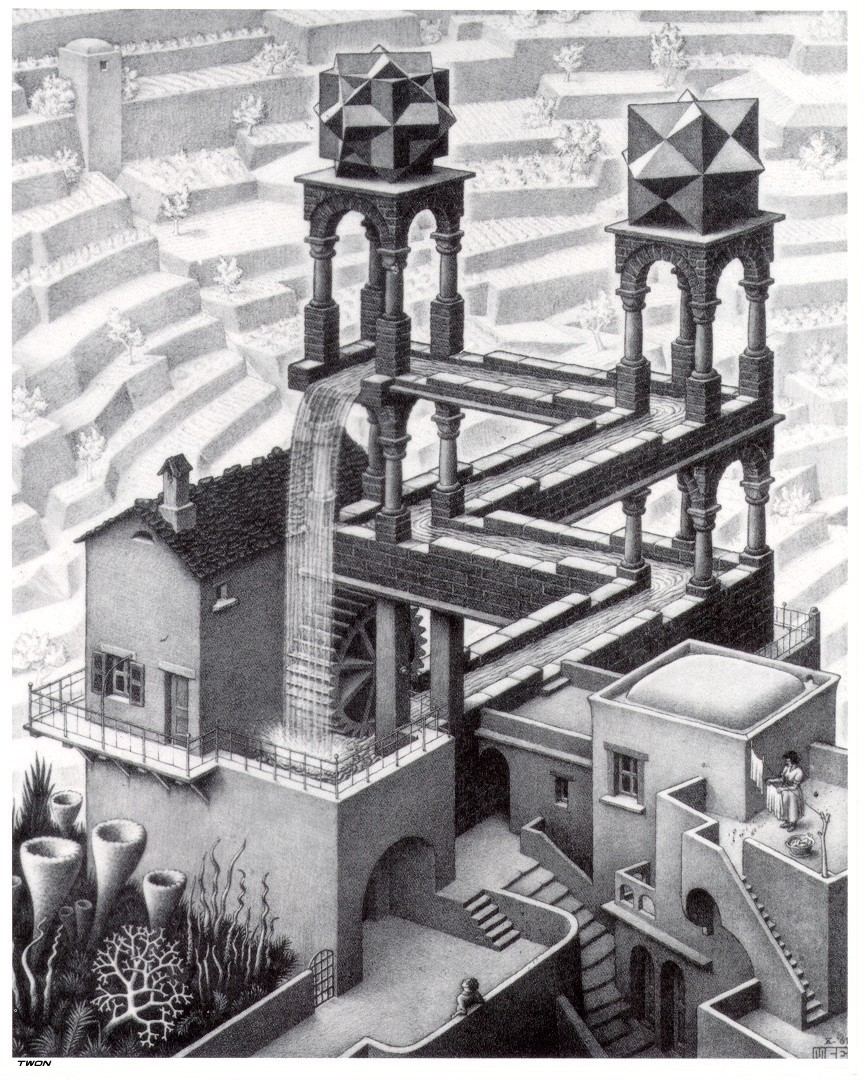# GS019: The Limits of Computation

### Meeting Time:

Mon 12-1 in CIT 506,
Wed & Fri 3-4 in the CIT 4th floor library

### Course Description:

Syllabus
Proposal (doc)
Lecture Schedule(ps)

### Lectures:

• Lecture 1: Introduction, Turing Machine (kmmatule, coming soon)
• Lecture 2: More TMs (kmmatule, draft) dvi ps pdf
• Lecture 3: Decidability (scanon, half-done):pdf
• Lecture 4: Undecidability and PCP (dpierce):html doc
• Lecture 5: Self reference and Recursion Theorem (bmares, erachlin): dvi ps pdf
• Lecture 6: Introduction to Complexity theory (jalper): pdf
• Lecture 7: NP completeness (ewbaer):
• Lecture 8: PSPACE completeness (msbean): doc
• Lecture 9: Diagonalization and Space/Time Hierarchy Theorems dvi ps pdf
• Lecture 10: Alternation (apienaar):
• Lecture 11: IP=PSPACE (bmares): jpg
• Lecture 12: Zero-Knowledge & PCP (apienaar,erachlin)

### Homeworks:

• HW1: Design a TM that decides {0n1n| n >= 0}, do 3.10-3.13 (one of), 3.16,3.19
Solutions (dvi, ps, pdf)
• HW2: Do exercises 4.15, 5.17, 5.20
Solutions (dvi, ps, pdf)
• HW3:To be found here: dvi ps pdf
Solutions (dvi, ps, pdf)
• HW4: Do exercises 7.28 and 7.29. Additionally, convince yourself coNP = NP is not necessarily true.  Argue that if coNP is not equal to NP, then P is not equal to NP
Solutions (dvi, ps, pdf)
• HW5: Do exercises 7.34 and 8.11
Solutions (dvi, ps, pdf)
• HW6: Do exercises 8.13, 8.19, 8.20
Solutions (dvi, ps, pdf)
• HW7: Do exercises 9.13, 9.11
Solutions (dvi, ps, pdf)
• HW8: Do exercises 10.12, and 10.14
Solutions (dvi, ps, pdf)
• HW9: Do exercises 10.11, and one of 10.15 & 10.16
(for 10.16, note the errata in Sipser)
Solutions (dvi, ps, pdf)